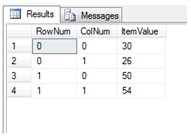# SQL Server MMULT function

MMULTN

Updated: 17 August 2010

Use MMULTN to calculate the matrix product of two arrays. The result is a TABLE where the maximum row number is equal to the number of rows in the first array and the maximum column number is the number of columns in the second array

For matrices not in normalized form, use the MMULT function.
Syntax
SELECT * FROM [wctMath].[wct].[MMULTN] (
<@Matrix_A_TableName, nvarchar(4000),>
,<@Matrix_A_Key1ColumnName, nvarchar(4000),>
,<@Matrix_A_Key2ColumnName, nvarchar(4000),>
,<@Matrix_A_DataColumnName, nvarchar(4000),>
,<@Matrix_A_GroupedColumnName, nvarchar(4000),>
,<@Matrix_A_GroupedColumnValue, sql_variant,>
,<@Matrix_B_TableName, nvarchar(4000),>
,<@Matrix_B_Key1ColumnName, nvarchar(4000),>
,<@Matrix_B_Key2ColumnName, nvarchar(4000),>
,<@Matrix_B_DataColumnName, nvarchar(4000),>
,<@Matrix_B_GroupedColumnName, nvarchar(4000),>
,<@Matrix_B_GroupedColumnValue, sql_variant,>)
Arguments
@Matrix_A_TableName
the name, as text, of the table or view that contains the values in the first or ‘A’ array to be used in the MMULT calculation.
@Matrix_A_Key1ColumnName
the name, as text, of the column in the table or view specified by @Matrix_A_TableName that contains the ‘row number’ value used in the array.
@Matrix_A_Key2ColumnName
the name, as text, of the column in the table or view specified by @Matrix_A_TableName that contains the ‘column number’ value used in the array.
@Matrix_A_DataColumnName
The name, as text, of the column in the table or view specified by @Matrix_A_TableName that contains the matrix values to be used in the product. Data returned from @Matrix_A_DataColumnName must be of the type float or of a type that implicitly converts to float.
@Matrix_A_GroupedColumnName
the name, as text, of the column in the table or view specified by @Matrix_A_TableName which will be used for grouping the results.
@Matrix_A_GroupedColumnValue
the column value to do the grouping on.
@Matrix_B_TableName
the name, as text, of the table or view that contains the values in the second or ‘B’ array to be used in the MMULT calculation.
@Matrix_B_Key1ColumnName
the name, as text, of the column in the table or view specified by @Matrix_B_TableName that contains the ‘row number’ value used in the array.
@Matrix_B_Key2ColumnName
the name, as text, of the column in the table or view specified by @Matrix_B_TableName that contains the ‘column number’ value used in the array.
@Matrix_B_DataColumnName
The name, as text, of the column in the table or view specified by @Matrix_B_TableName that contains the matrix values to be used in the product. Data returned from @Matrix_B_DataColumnName must be of the type float or of a type that implicitly converts to float.
@Matrix_B_GroupedColumnName
the name, as text, of the column in the table or view specified by @Matrix_B_TableName which will be used for grouping the results.
@Matrix_B_GroupedColumnValue
the column value to do the grouping on.
Return Types
TABLE (
[RowNum] [int] NULL,
[ColNum] [int] NULL,
[ItemValue] [float] NULL
Remarks
·         The number of columns in the ‘A’ array must be equal to the number of rows in the ‘B’ array or an error will be returned.
·         Use the MMULTN_q function for more complex queries or to pass the matrices directly into the function without having to store them in a table.
·         Use MMULT for a table not in normal form.
·         If the array contains NULL, then NULL will be returned.
·         If the array contains a blank, it will be treated as zero.
·         The function returns an error if either array contains a non-numeric value.
Examples
CREATE TABLE #m (
MatrixID    varchar(5),
rowno       int,
colno       int,
val         float
)

INSERT INTO #M VALUES('2A',0,0,2)
INSERT INTO #M VALUES('2A',0,1,4)
INSERT INTO #M VALUES('2A',1,0,8)
INSERT INTO #M VALUES('2A',1,1,6)
INSERT INTO #M VALUES('2B',0,0,1)
INSERT INTO #M VALUES('2B',0,1,3)
INSERT INTO #M VALUES('2B',1,0,7)
INSERT INTO #M VALUES('2B',1,1,5)

SELECT *
FROM wct.MMULTN(
'#m'
,'rowno'
,'colno'
,'val'
,'MATRIXID'
,'2A'
,'#m'
,'rowno'
,'colno'
,'val'
,'MATRIXID'
,'2B'
)

This produces the following result.Copyright 2008-2021 Westclintech LLC         Privacy Policy        Terms of Service